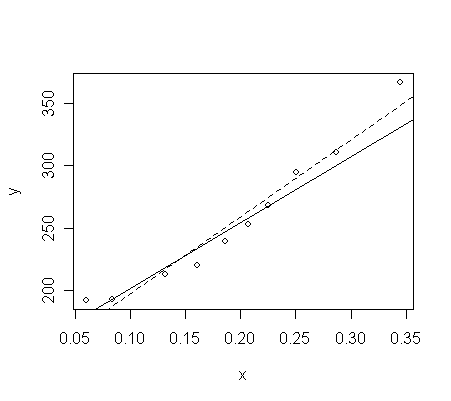# Weighted least square (Reading: Faraway(2005, 1st edition), 6.2)

Look at here for a description of the data that we will use in this section.

• Q: Can you figure out from the description what weights should be used for the data and why?

> p <- read.table("phy.data", header=T) # note the extra argument to cope with not having row labels
> p # take a look of the data

momentum energy crossx sd

1         4  0.345    367 17

2         6  0.287    311  9

3         8  0.251    295  9

...deleted...

10      150  0.060    192  5

Notice that

• The sd decreases along with the reduction of energy.

• Since the weights should be inversely proportional to the variance, this suggests that the weights should be set to 1/sd2.

> x <- p[, 2] # extract the energy variable, the predictor
> y <- p[, 3] # extract the crossx variable, the response
> w <- 1/p[, 4]^2 # the weights
> g <- lm(y~x, weights=w) # fit the model with w as weights. "weights" adds as an option.
> summary(g) # take a look of the results

Call:

lm(formula = y ~ x, weights = w)

Residuals:

Min         1Q     Median         3Q        Max

-2.323e+00 -8.842e-01  1.266e-06  1.390e+00  2.335e+00

Coefficients:

Estimate Std. Error t value Pr(>|t|)

(Intercept)  148.473      8.079   18.38 7.91e-08 ***

x            530.835     47.550   11.16 3.71e-06 ***

---

Signif. codes:  0 '***' 0.001 '**' 0.01 '*' 0.05 '.' 0.1 ' ' 1

Residual standard error: 1.657 on 8 degrees of freedom

Multiple R-Squared: 0.9397,     Adjusted R-squared: 0.9321

F-statistic: 124.6 on 1 and 8 DF,  p-value: 3.710e-06

• We can test the significance of x by looking at the p-value from the coefficients table or by looking at the overall F-stat (they are equivalent, 11.162=124.6, because this is a simple regression case).

• But, if we want to do it the hard way, we must construct the analysis of variance table by hand as follows:

> sum(w*g\$res*g\$res) # the residual sum of squares

 21.95265

> wm <- sum(w*y)/sum(w) # the weighted mean of y
> sum(w*(y-wm)*(y-wm)) # the weighted total SS

 363.944

> 363.944 - 21.95265 # the regression SS

 341.9914

> (341.9914/1)/(21.95265/8) # the overall F statistic

 124.6287

Let's try fitting the regression without weights and see what the difference is:

> g1 <- lm(y~x) # fit the model without weights
> summary(g1) # take a look of the fitted model

Call:

lm(formula = y ~ x)

Residuals:

Min      1Q  Median      3Q     Max

-14.773  -9.319  -2.829   5.571  19.818

Coefficients:

Estimate Std. Error t value Pr(>|t|)

(Intercept)   135.00      10.08   13.40 9.21e-07 ***

x             619.71      47.68   13.00 1.16e-06 ***

---

Signif. codes:  0 '***' 0.001 '**' 0.01 '*' 0.05 '.' 0.1 ' ' 1

Residual standard error: 12.69 on 8 degrees of freedom

Multiple R-Squared: 0.9548,     Adjusted R-squared: 0.9491

F-statistic: 168.9 on 1 and 8 DF,  p-value: 1.165e-06

• Compare the output to the summary of model g. Explain their differences.

• R-square: why would g1 has a higher value than g?

• σ-hat: Why are they so different? Do they estimate the same parameter?

• residuals: Why are their ranges so different?

• The two fits can be compared:

> plot(x,y) # scatter plot of x and y
> abline(g) # the fitted line of model g, solid line
> abline(g1, lty=2) # the fitted line of model g2, dashed line• The un-weighted fit (dashed line) appears to fit the data better overall

• But remember that for lower values of energy, the variance in the response is less.

• The weighted fit (solid line) tries to catch these points with lower energy better than the others.

Weighted least square can be regarded as regressing sqrt(wi)xi on sqrt(wi)yi using ordinary least square:

> ym<-sqrt(w)*y # calculate sqrt(wi)yi
> xm<-sqrt(w)*x  # calculate sqrt(wi)xi

> g2 <- lm(ym~sqrt(w)+xm-1) # regressing sqrt(wi)xi on sqrt(wi)yi. "-1" drop the intercept.

• Note that the 1 column in X-matrix is replaced by sqrt(wi)

> summary(g2) # take a look of the results

Call:

lm(formula = ym ~ sqrt(w) + xm - 1)

Residuals:

Min         1Q     Median         3Q        Max

-2.323e+00 -8.842e-01  1.266e-06  1.390e+00  2.335e+00

Coefficients:

Estimate Std. Error t value Pr(>|t|)

sqrt(w)  148.473      8.079   18.38 7.91e-08 ***

xm       530.835     47.550   11.16 3.71e-06 ***

---

Signif. codes:  0 '***' 0.001 '**' 0.01 '*' 0.05 '.' 0.1 ' ' 1

Residual standard error: 1.657 on 8 degrees of freedom

Multiple R-Squared: 0.9983,     Adjusted R-squared: 0.9978

F-statistic:  2303 on 2 and 8 DF,  p-value: 9.034e-12

• Compare the output to the summary of model g. What quantities are different? Explain why.

• To get the correct overall F-test, we can use:

> anova(lm(ym~sqrt(w)-1), g2) # the correct F-statistics

Analysis of Variance Table

Model 1: ym ~ sqrt(w) - 1

Model 2: ym ~ sqrt(w) + xm - 1

Res.Df    RSS Df Sum of Sq      F    Pr(>F)

1      9 363.94

2      8  21.95  1    341.99 124.63 3.710e-06 ***

---

Signif. codes:  0 '***' 0.001 '**' 0.01 '*' 0.05 '.' 0.1 ' ' 1

• You can use the two RSSs in the correct F-statistic to obtain the right R-square (How?)

Let's also check their residuals.

> print(g\$res, digits=2) # the residuals of model g

1       2       3       4       5       6       7       8       9      10

35.389  10.177  13.287   0.089  -5.356  -8.209 -13.938  -5.544  -0.063  11.677

> print(g2\$res, digits=2) # the residuals of model g2

1      2      3      4      5      6      7      8      9     10

2.082  1.131  1.476  0.013 -0.765 -1.368 -2.323 -0.924 -0.013  2.335

• Why are the two groups of residuals so different?

• Which residuals is yi minus yi-hat? Which residuals is sqrt(wi)yi minus sqrt(wi)yi-hat?

• Which residuals can be used to perform diagnostics (i.e., residual analysis) and to calculate σ-hat by directly taking sum of their squares?

• Let's find out the relationship between the two groups of residuals:

> print(sqrt(w)*g\$res, digits=2) # check if it equals residuals of model g2

1      2      3      4      5      6      7      8      9     10

2.082  1.131  1.476  0.013 -0.765 -1.368 -2.323 -0.924 -0.013  2.335